The desirable performance of many systems today depends on their thermal management system. For instance, a gas turbine can reach temperatures of up to 800° C. Without a proper thermal management system, some turbine components will deform and fail due to heat. This is one of the reasons why gas turbine blades are designed to have multiple airfoils through which cooling air enters to maintain a stable thermal environment.

This mode of heat transfer is called convection and is also the principle guiding the operation of industrial heat exchangers and heat sinks in electronics. However, while cooling air enhances heat transfer, several other factors contribute to the performance of cooling air for heat transfer enhancement. One of these is turbulence.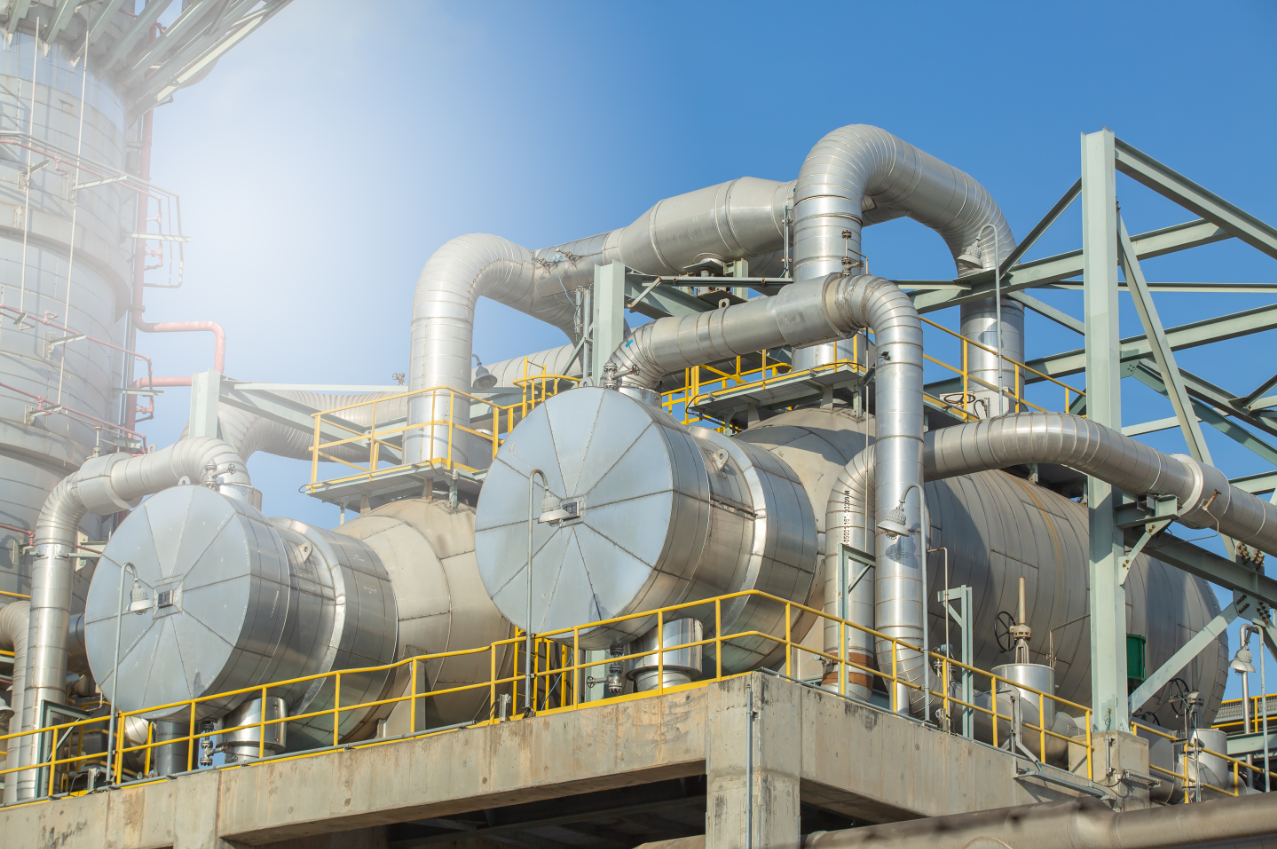Figure 1: The desirable performance of many systems today depends on their thermal management system. Source: tong2530/Adobe Stock

## What is turbulence?

Turbulence describes chaotic eddies (or disorderly movement) of air disturbed from a calmer state (laminar) by excessive kinetic energy. To better understand turbulent flows, consider Figure 2, which shows a turbulent airflow in a pipe.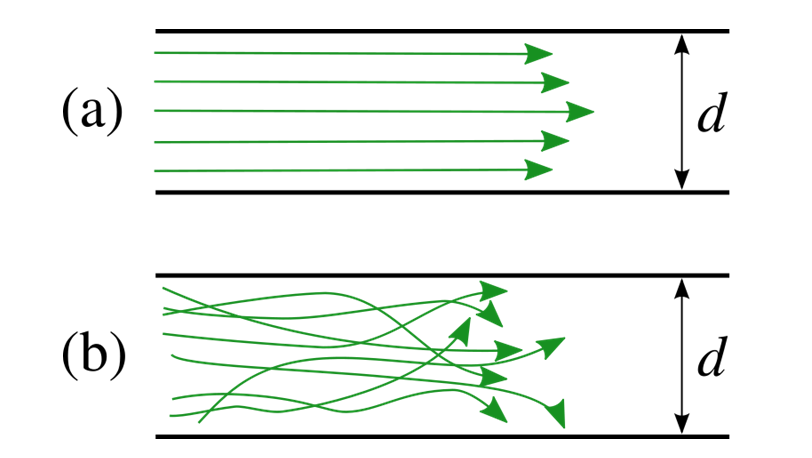Figure 2: Turbulent flow is characterized by the disorderly movement of the fluid.

As shown in the figure above, we say a flow is turbulent if the fluid particles move in a zig-zag manner or if the fluid undergoes irregular fluctuations and mixing. In turbulent flow, the velocity of the fluid at any point changes in magnitude and direction. For example, ocean currents and flow in pumps and turbines are generally turbulent.

While all turbulent flows are chaotic in nature, not all chaotic flows are turbulent. The turbulence level of a fluid is usually predicted using a dimensionless parameter called the Reynolds number.

## Predicting turbulence level using the Reynolds number

The Reynolds number represents the ratio of internal forces to viscous forces of a fluid. It is defined as: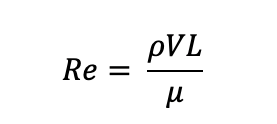Equation 1: Reynolds number equation for measuring turbulence.

Where:

p = density of the fluid (kg/m3)

V = velocity of the fluid with respect to the object

L = length of fluid flow

u = dynamic viscosity (kg/ms)

Fluid flows with a high Reynolds number (typically greater than 5,000) are typically turbulent, while those with a lower Reynolds number are laminar. Nevertheless, heat transfer rate (and heat transfer coefficient) is usually more significant under turbulent flow conditions.

## Heat transfer coefficient

Consider a hot metal tube through which air flows to cool the tube, as shown in Figure 3.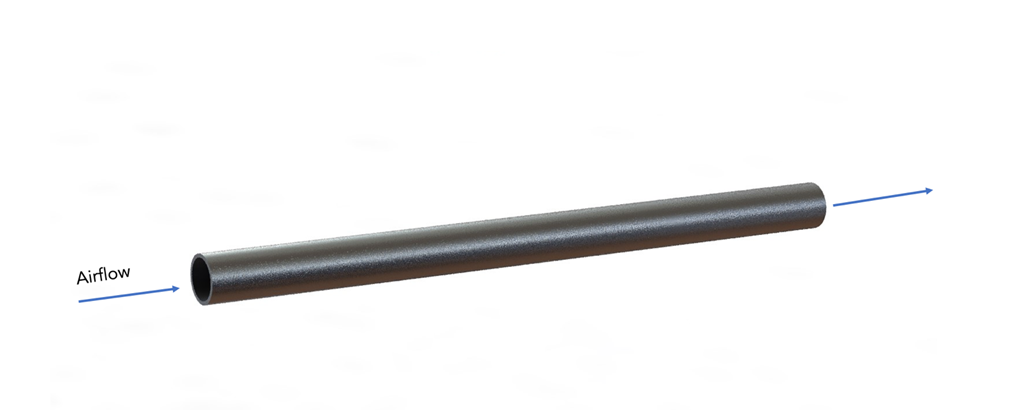Figure 3: Cooling of a metal tube using airflow.

Engineers would agree that the rate of heat flow from the metal to the air is affected by the tube’s thermal conductivity, thickness and surface area. However, these factors are associated with only the properties of the metal tube. In reality, the heat transfer rate is also affected by fluid flow.

The heat transfer coefficient is a parameter that combines the property of the fluid flow and solid geometry to estimate heat transfer (typically by convection). It can be calculated using: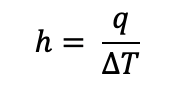Equation 2: Heat transfer coefficient equation for turbulent fluids.

Where:

h = heat transfer coefficient

q = local heat flux density (W/m2)

ΔT = temperature difference (K)

Engineers can achieve a better heat transfer performance by simply increasing the system’s heat transfer coefficient. And one way of increasing the heat transfer coefficient is by enhancing the flow turbulence.

## How does flow turbulence enhances heat transfer coefficient?

As the Reynolds number formula suggests, flow turbulence is typically achieved at higher fluid velocities. At high Reynolds numbers (in the turbulent regime), there is substantial breaking away of the fluid from the tube wall. This causes significant mixing of the boundary layer and the bulk fluid, enhancing the heat and momentum transfer between the fluid particles.

For this reason, engineers usually opt for the forced convection technique when creating cooling systems for electronics and other devices. In forced convection, the fluid motion is generated by an external source (like fans, suction devices and compressors), allowing higher flow velocities and turbulence. As such, this fluid transport enhances the heat transfer performance.

## Increasing heat transfer performance using rib turbulators

Rib turbulators are solid obstructions placed in a flow channel at different intervals. These turbulators come in different geometries (like cuboid, trapezoidal and cylindrical). They are designed to create (and maintain turbulent flow), improving heat transfer performance.

To better understand how rib turbulators work, consider Figure 4, which shows the section of a metal tube with turbulators over which air flows.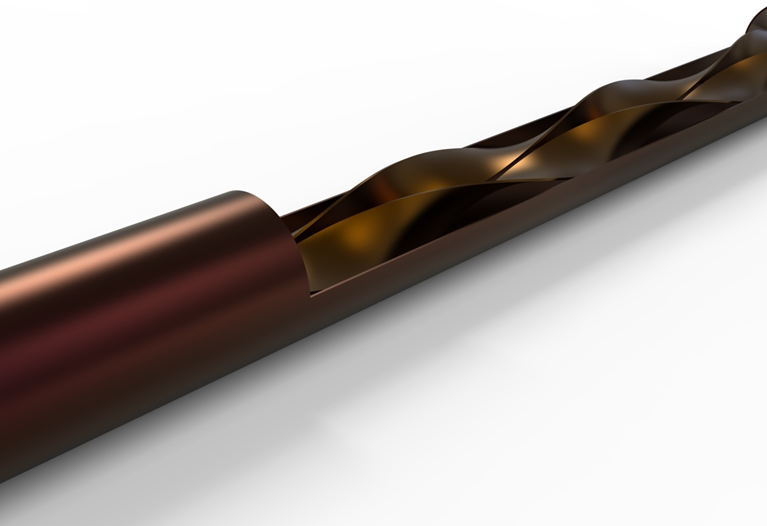Figure 4: Metal tube with twisted tape turbulators.

Not only do these turbulators increase the heat transfer surface area, but they also break up the viscous sublayer of the flow. This operation increases flow turbulence and fluid mixing, improving the heat transfer performance.

However, because these turbulators disturb the fluid path, they typically cause pressure drops. As a result, more pumping power may be required to attain desirable flow performance. Therefore, engineers are advised to perform a Performance Evaluation Criteria (PEC) to compare the combined effect of heat transfer enhancement and pressure drop when designing thermal management systems for a device.